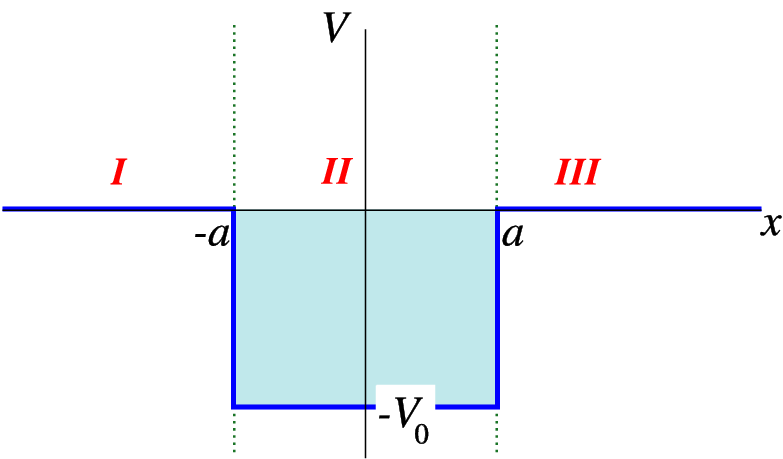## Chapter 4 Bound states of the square well

One of the simplest potentials to study the properties of is the so-called square well potential,

 $V=\left\{\begin{array}{cc}0\hfill & |x|>a\hfill \\ -{V}_{0}\hfill & |x| (4.1)Figure 4.1: The square well potential

We deﬁne three areas, from left to right I, II and III. In areas I and III we have the Schrödinger equation

 $-\frac{{\hslash }^{2}}{2m}\frac{{d}^{2}}{d{x}^{2}}\psi \left(x\right)=E\psi \left(x\right)$ (4.2)

whereas in area II we have the equation

 $-\frac{{\hslash }^{2}}{2m}\frac{{d}^{2}}{d{x}^{2}}\psi \left(x\right)=\left(E+{V}_{0}\right)\psi \left(x\right)$ (4.3)

__________________________________________________________________

Solution to a few ODE’s

. In this class we shall quite often encounter the ordinary diﬀerential equations

 $\frac{{d}^{2}}{d{x}^{2}}f\left(x\right)=-{\alpha }^{2}f\left(x\right)$ (4.4)

which has as solution

 $f\left(x\right)={A}_{1}cos\left(\alpha x\right)+{B}_{1}sin\left(\alpha x\right)={C}_{1}{e}^{i\alpha x}+{D}_{1}{e}^{-i\alpha x},$ (4.5)

and

 $\frac{{d}^{2}}{d{x}^{2}}g\left(x\right)=+{\alpha }^{2}g\left(x\right)$ (4.6)

which has as solution

 $g\left(x\right)={A}_{2}cosh\left(\alpha x\right)+{B}_{2}sinh\left(\alpha x\right)={C}_{2}{e}^{\alpha x}+{D}_{2}{e}^{-\alpha x}.$ (4.7)
________________________________________________________________________________________________________

Let us ﬁrst look at $E>0$. In that case the equation in regions $I$ and $III$ can be written as

 $\frac{{d}^{2}}{d{x}^{2}}\psi \left(x\right)=-\frac{2m}{{\hslash }^{2}}E\psi \left(x\right)=-{k}^{2}\psi \left(x\right),$ (4.8)

where

 $k=\sqrt{\frac{2m}{{\hslash }^{2}}E}.$ (4.9)

The solution to this equation is a sum of sines and cosines of $kx$, which cannot be normalised: Write ${\psi }_{III}\left(x\right)=Acos\left(kx\right)+Bsin\left(kx\right)$( $A$, $B$, complex) and calculate the part of the norm originating in region $III$,

$\begin{array}{rcll}{\int }_{a}^{\infty }|\psi \left(x\right){|}^{2}dx& =& {\int }_{a}^{\infty }|A{|}^{2}{cos}^{2}kx+|B{|}^{2}{sin}^{2}kx+2\Re \left(A{B}^{\ast }\right)sin\left(kx\right)cos\left(kx\right)dx& \text{}\\ & =& \underset{N\to \infty }{lim}N{\int }_{a}^{2\pi ∕k}|A{|}^{2}{cos}^{2}\left(kx\right)+|B{|}^{2}{sin}^{2}\left(kx\right)& \text{}\\ & =& \underset{N\to \infty }{lim}N\left(|A{|}^{2}∕2+|B{|}^{2}∕2\right)=\infty .& \text{(4.10)}\text{}\text{}\end{array}$

We also ﬁnd that the energy cannot be less than $-{V}_{0}$, since we vannot construct a solution for that value of the energy. We thus restrict ourselves to $-{V}_{0}. We write

 $E=-\frac{{\hslash }^{2}{k}^{2}}{2m},\phantom{\rule{2.77695pt}{0ex}}\phantom{\rule{2.77695pt}{0ex}}\phantom{\rule{2.77695pt}{0ex}}E+{V}_{0}=\frac{{\hslash }^{2}{\kappa }^{2}}{2m}.$ (4.11)

The solutions in the areas I and III are of the form ( $i=1,3$)

 $\psi \left(x\right)={A}_{i}{e}^{kx}+{B}_{i}{e}^{-kx}.$ (4.12)

In region II we have the oscillatory solution

 $\psi \left(x\right)={A}_{2}cos\left(\kappa x\right)+{B}_{2}sin\left(\kappa x\right).$ (4.13)

Now we have to impose the conditions on the wave functions we have discussed before, continuity of $\psi$ and its derivatives. Actually we also have to impose normalisability, which means that ${B}_{1}={A}_{3}=0$(exponentially growing functions can not be normalised). As we shall see we only have solutions at certain energies. Continuity implies that

$\begin{array}{rcll}{A}_{1}{e}^{-ka}+{B}_{1}{e}^{ka}& =& {A}_{2}cos\left(\kappa a\right)-{B}_{2}sin\left(\kappa a\right)& \text{}\\ {A}_{3}{e}^{ka}+{B}_{3}{e}^{-ka}& =& {A}_{2}cos\left(\kappa a\right)+{B}_{2}sin\left(\kappa a\right)& \text{}\\ k{A}_{1}{e}^{ka}-k{B}_{1}{e}^{ka}& =& \kappa {A}_{2}sin\left(\kappa a\right)+\kappa {B}_{2}cos\left(\kappa a\right)& \text{}\\ k{A}_{3}{e}^{ka}-k{B}_{3}{e}^{-ka}& =& -\kappa {A}_{2}sin\left(\kappa a\right)+\kappa {B}_{2}cos\left(\kappa a\right)& \text{(4.14)}\text{}\text{}\end{array}$

Tactical approach: We wish to ﬁnd a relation between $k$ and $\kappa$(why?), removing as manby of the constants $A$ and $B$. The trick is to ﬁrst ﬁnd an equation that only contains ${A}_{2}$ and ${B}_{2}$. To this end we take the ratio of the ﬁrst and third and second and fourth equation:

$\begin{array}{rcll}k& =& \frac{\kappa \left[{A}_{2}sin\left(\kappa a\right)+{B}_{2}cos\left(\kappa a\right)\right]}{{A}_{2}cos\left(\kappa a\right)-{B}_{2}sin\left(\kappa a\right)}& \text{}\\ k& =& \frac{\kappa \left[{A}_{2}sin\left(\kappa a\right)-{B}_{2}cos\left(\kappa a\right)\right]}{{A}_{2}cos\left(\kappa a\right)+{B}_{2}sin\left(\kappa a\right)}& \text{(4.15)}\text{}\text{}\end{array}$

We can combine these two equations to a single one by equating the right-hand sides. After deleting the common factor $\kappa$, and multiplying with the denominators we ﬁnd

$\begin{array}{rcll}\left[{A}_{2}cos\left(\kappa a\right)+{B}_{2}sin\left(\kappa a\right)\right]\left[{A}_{2}sin\left(\kappa a\right)-{B}_{2}cos\left(\kappa a\right)\right]& & & \text{}\\ & =& \left[{A}_{2}sin\left(\kappa a\right)+{B}_{2}cos\left(\kappa a\right)\right]\left[{A}_{2}cos\left(\kappa a\right)-{B}_{2}sin\left(\kappa a\right)\right],& \text{(4.16)}\text{}\text{}\end{array}$

which simpliﬁes to

 ${A}_{2}{B}_{2}=0$ (4.17)

We thus have two families of solutions, those characterised by ${B}_{2}=0$ and those that have ${A}_{2}=0$.